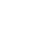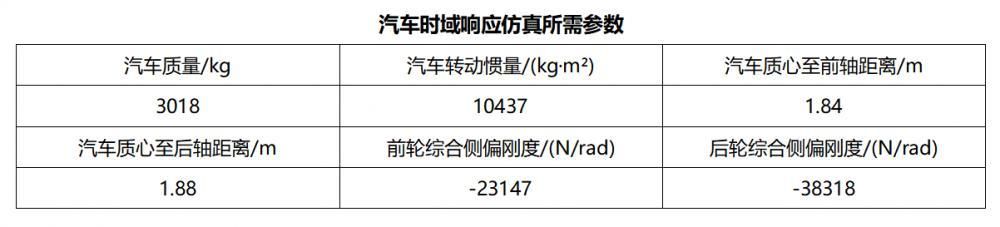G1(s) = ((s - a11)*b21 + a21*b11) / s²- (a11 + a22)*s + a11*a22 - a12*a21;

G2(s) = ((s - a22)*b11 + a12*b21) / s²- (a11 + a22)*s + a11*a22 - a12*a21;

a11 = (Ka1 + Ka2) / mu;     a12 = (aKa1 - bKa2 - mu²) / mu²;

a21 = (aKa1 - bKa2) / Iz;     a22 = (a²Ka1 + b²Ka2) / Iz*u;

b11 = -Ka1 / mu;            b21 = -aKa1/Iz；

### 汽车横摆角度速度传递函数

>> m=3018; Iz=10437; a=1.84; b=1.88; k1=-23147; k2=-38318;

>> u= [10 20 30];

>> a11 = (k1 + k2)/m./u;

>> a12 = (a*k1 - b*k2 -m.*u^2)/m./u^2;

>> a21 = (a * k1 - b * k2)/Iz;

>> a22=(a^2*k1 + b^2*k2)/Iz./u;

>> b11 = -k1/m./u;

>> b21 = -a*k1/Iz;

>> b1 = b21;

>> b2 = a21*b11-a11*b21;

>> b3 = -a11-a22;

>> b4 = a11.*a22-a12.*a21;

>> num = [b1 b2];

>> den = [1,b3,b4];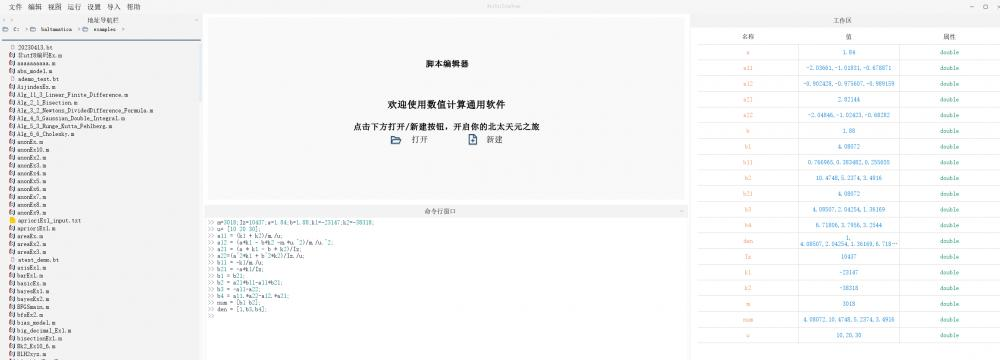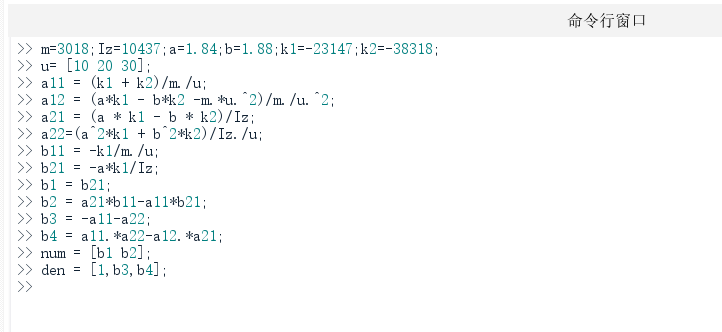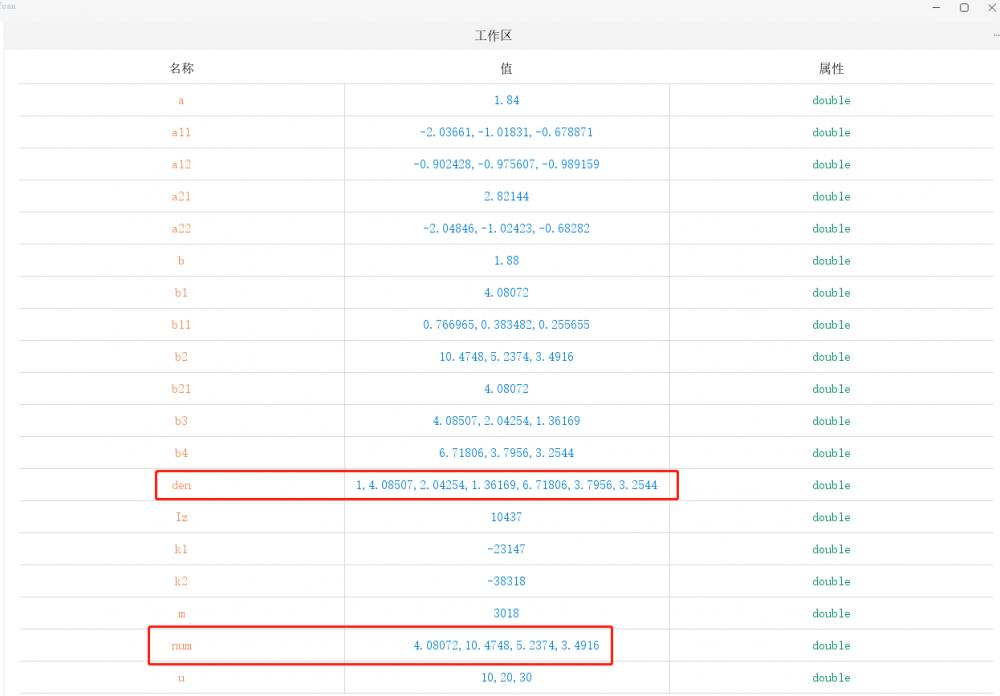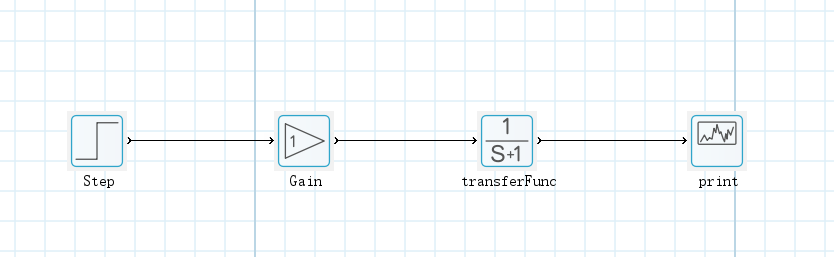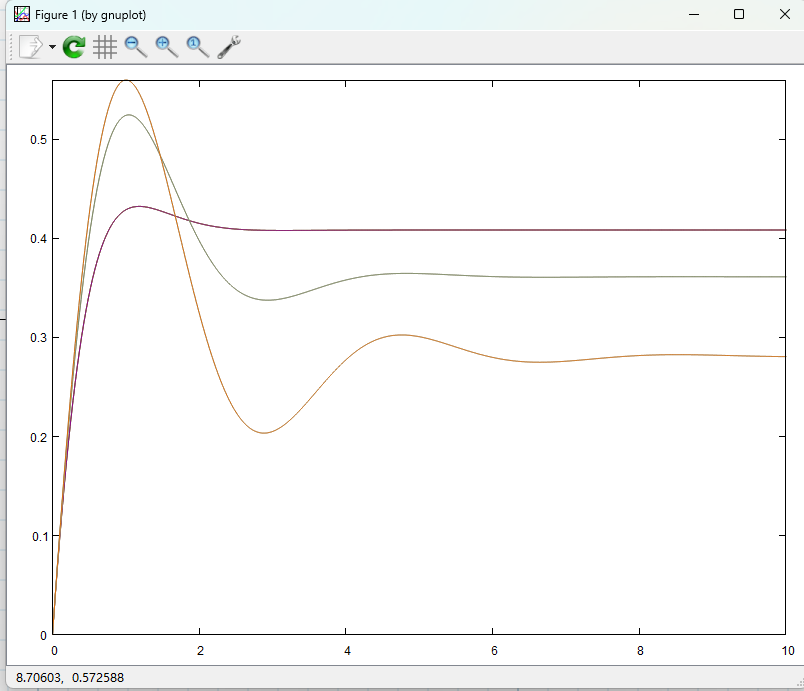### 质心偏侧角传递函数

>> m=3018;Iz=10437;a=1.84;b=1.88;k1=-23147;k2=-38318;

>> u= [10 20 30];

>> a11 = (k1 + k2)/m./u;

>> a12 = (a*k1 - b*k2 -m.*u.^2)/m./u.^2;

>> a21 = (a * k1 - b * k2)/Iz;

>> a22=(a^2*k1 + b^2*k2)/Iz./u;

>> b11 = -k1/m./u;

>> b21 = -a*k1/Iz;

>> b1 = b11;

>> b2 = a12*b21-a22*b11;

>> b3 = -a11-a22;

>> b4 = a11.*a22-a12.*a21;

>> num = [b1 b2];

>> den = [1,b3,b4];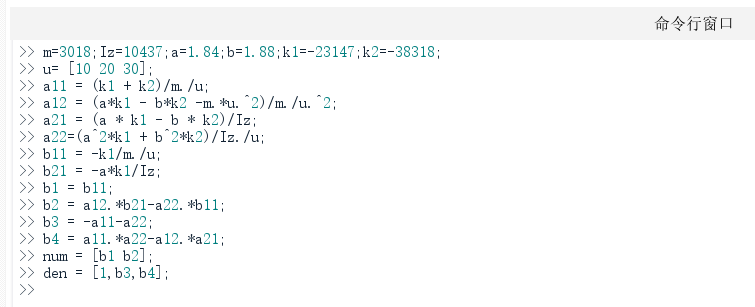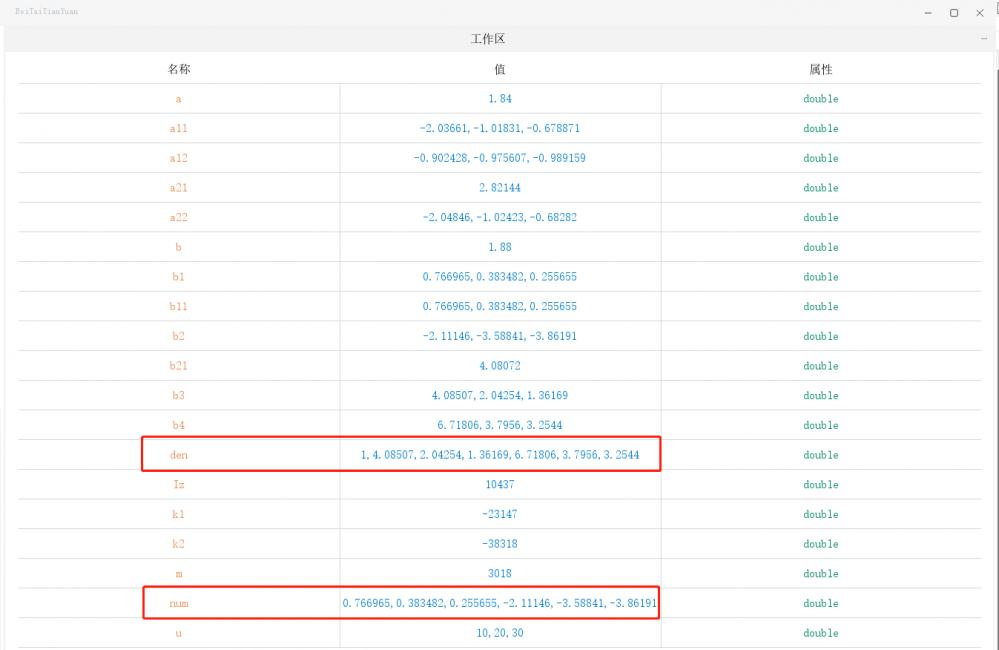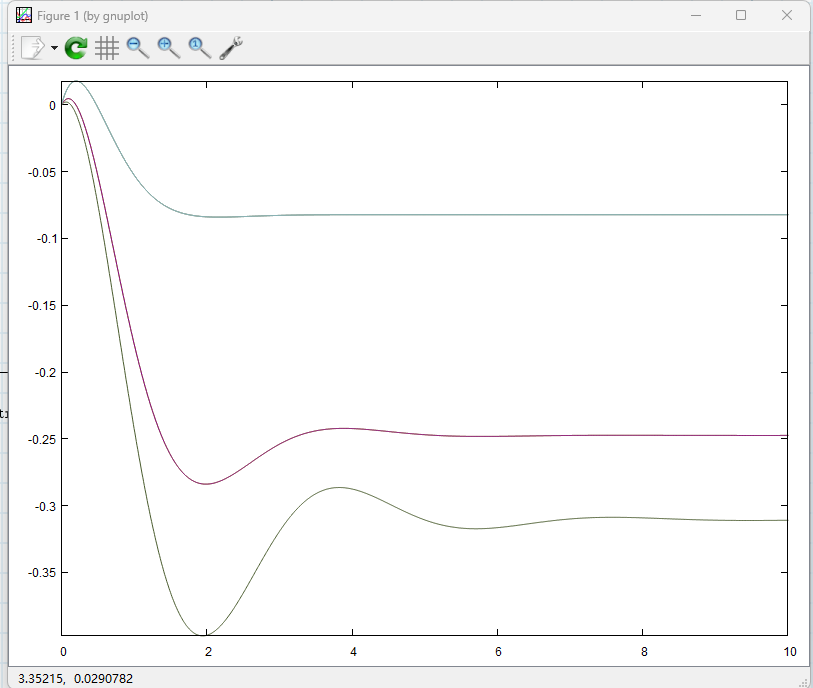343 0 0 收藏 回复

## 回复

### 社区牛人